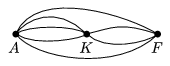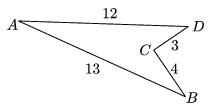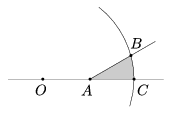Mathematical and Physical Journal
for High Schools
Issued by the MATFUND Foundation
 Already signed up? New to KöMaL?

# New exercises and problems in MathematicsNovember 2004## New exercises for beginners

Solutions can be submitted only by students of grade 9. Maximum score for each exercise (sign "K") is 6 points.

K. 13. Determine the last two digits of the sum 71+72+...+72005.

K. 14. There are several roads connecting three towns Downton, Upton and Middleton. The number of direct roads between any pair of towns is at least 3 and at most 10. There are 33 different paths from Downton to Upton, including roads that lead there directly as well as paths passing through Middleton. Similarly, one can go from Middleton to Upton directly or through Downton in 23 different ways. Given the information above, how many different paths are there altogether from Middleton to Downton (including direct roads as well as paths passing through Upton)? Example: In the Figure there are 2 direct roads from Downton (A) to Uptown (F) and there are 3.2=6 paths through Middleton (K), which make 8 paths altogether.K. 15. The convex quadrilateral ABCD has an angle of 100o at vertex A. Given that the diagonal AC divides the quadrilateral into an equilateral triangle and an isosceles triangle, calculate the measures of the interior angles of the quadrilateral.

K. 16. a) Using each of the digits 9, 8, 7, 6 once, form two two-digit numbers such that their product is maximal. b) Using each of the digits 9, 8, 7, 6, 5, 4 once, form three two-digit numbers such that their product is maximal. Give reasons for your answer.

K. 17. The sides of the concave quadrilateral ABCD are AB=13 cm, BC=4 cm, CD=3 cm, DA=12 cm, and its interior angle at vertex C is 270o. Find the area of the quadrilateral.K. 18. Determine the four distinct digits a, b, c and d, such that $\displaystyle \overline{abcd}:\overline{dcba}=4$, where $\displaystyle \overline{abcd}$ and $\displaystyle \overline{dcba}$ denote four-digit numbers.

K. 12. For how many positive numbers n is it true that 2004n is a factor of 2004! ? [2004! denotes the product of integers from 1 to 1024.] (Suggested by Á. Englert, Zalaegerszeg.)## New exercises

Maximum score for each exercise (sign "C") is 5 points.

C. 780. There were three problems posed in a mathematics competition. The first problem was solved by 85 percent of the participants, the second one was solved by 80 percent and the last one by 75 percent. Prove that at least 40 percent solved all three problems.

C. 781. Find the positive primes p>q>r, such that p2- (q+r)2=136.

C. 782. A sailboat is travelling parallel to the shore of the lake Balaton, at a distance of 200 metres. A swimmer wants to reach the approaching sailboat by moving along a straight line. At what angle to the shore should he leave if the speed of the boat is 15 km/h, the speed of the swimmer is 2 km/h, and at start his distance from the point of the shore that is closest to the boat is 2 km? (Suggested by L. Koncz, Budapest)

C. 783. The shaded region in the figure is bounded by the arms of the 30o angle of vertex A and a circular arc centred at the point O. Find the area of the region, given that AO=AB=1.

C. 784. In the cuboid ABCDEFGH (with vertices labelled in the conventional way), AE= 1, AD = 2, AB =3. Find the volume of the solid whose vertices are A, C, and the midpoints of the edges around the face EFGH.## New problems

The maximum scores for problems (sign "B") depend on the difficulty. It is allowed to send solutions for any number of problems, but your score will be computed from the 6 largest score in each month.

B. 3762. Two cylindrical tanks were filled with water. At 12 noon, two pumps of the same power started to pump the water, one from each tank, at a constant rate. At 2 p.m. the water levels were the same. At 5 p.m. the first tank was finished, and at 8 p.m. the second tank became empty, too. If the height of the second tank is 10 metres, how tall is the first one? (3 points)

B. 3763. P is a point in the interior of a convex quadrilateral ABCD. Prove that

PA2+PB2+PC2+PD2$\displaystyle \ge$2tABCD.

(3 points)

B. 3764. C1 divides the side AB of an equilateral triangle ABC in a ratio 1:3. (It lies closer to A.) The points A1, A2 and A3 divide the side BC into four equal parts. Find the sum of the angles AA1C1, AA2C1 and AA3C1. (4 points)

B. 3765. Given that the product of each pair out of 25 different positive integer not greater than 1000 is a perfect square, prove that the numbers themselves are also square numbers. (4 points)

B. 3766. There were four problems in a mathematics competition. The first problem was solved by 85 percent of the participants, the second one was solved by 80 percent, the third one was solved by 75 percent and the last one by 70 percent. What percentage of the participants, at least, must have solved all four problems? (4 points)

B. 3767. $\displaystyle \alpha$, $\displaystyle \beta$, $\displaystyle \gamma$ are the angles of a triangle. Given that sin $\displaystyle \alpha$+sin $\displaystyle \beta$=(cos $\displaystyle \alpha$+cos $\displaystyle \beta$)sin $\displaystyle \gamma$, determine the angle $\displaystyle \gamma$. (3 points)

B. 3768. A rectangle T0 is cut into two non-congruent but similar rectangles T1 and T1' with a line parallel to a side. The procedure is repeated for the resulting rectangle T1. Then it is repeated again for one of the parts obtained, and so on. Is there a rectangle T0, such that the procedure can be repeated to infinity? (5 points)

B. 3769. Given three tangents and one focus of an ellipse, construct by ruler and compass, the other focus. (4 points)

B. 3770. Into how many parts do the planes of the faces of a regular octahedron divide the space? (5 points)

B. 3771. Let $\displaystyle a_k=\frac{1}{\binom{n}{k}}$, $\displaystyle b_k=\frac{1}{2^{n-k}}$, k = 1,2,...,n. Prove that

$\displaystyle a_1+\frac{a_2}{2}+\frac{a_3}{3}+\dots+\frac{a_n}{n}=b_1+\frac{b_2}{2}+ \frac{b_3}{3}+\dots+\frac{b_n}{n}.$

(5 points)Maximum score for each advanced problem (sign "A") is 5 points.

A. 356. The sequence Pn(x) of polynomials is defined by the following recurrence: P0(x)=0, P1(x)=1 and Pn(x)=x.Pn-1(x)+(1-x).Pn-2(x). What are the roots of Pn(x)?

A. 357. k1,k2,k3, ... are disjoint circles. The radius of the circle ki is $\displaystyle \frac{1}{i}$ and its centre is Pi. Is it possible for the sequence of points Pi to be convergent?

A. 358. ab, c are positive numbers, such that abc=1. Prove that

$\displaystyle \frac{1}{a}+\frac{1}{b}+\frac{1}{c}-\frac{3}{a+b+c}\geq2\left( \frac{1}{a^2}+\frac{1}{b^2}+\frac{1}{c^2}\right)\cdot\frac{1}{a^2+b^2+c^2}.$